# Impedance fitting

When measuring components with a network analyzer, often is useful to fit a simple circuit model to the measured impedance.
The method described in  and  can be used to easily compute the equivalent circuit model parameters.
Below are some common equivalent circuits used, which are the same usually available on the Agilent Impedance Analyzers, and their recommended usage according to the Agilent User's Manual. The circuits impedance formula is also shown, both in its usual form and also in the form needed to apply the method described in the referenced articles.

## Fitting procedure overview

Given a generic impedance function written in polynomial formthe following error function can be definedwhere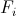are the measured impedance function values at the frequencies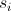.
By differentiating the error functionwith respect to all the unknown coefficientsand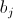and then setting all these derivatives to zero, a system of equations is obtained which allows to determine the unknown coefficients.
The matrix system has the canonical form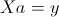where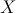is amatrix andandare vectors of length, where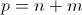.

The matrix system can be written using sub-matrices as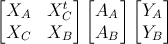where the sub-matrices and sub-vectors are defined as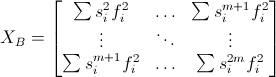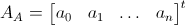The matrix equation can then be solved byIn practice two problems arise: one is thatis a complex matrix,is also a complex vector while we expectto be a real vector. The other problem is that the condition number ofis often very large, which can cause large errors when computing the coefficients.

## Circuit ATypical usage : inductor with high core losses

Impedance :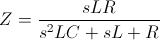Impedance function to be used for fitting :## Circuit B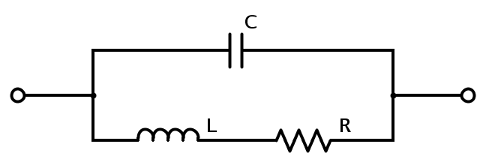Typical usage : inductor or resistor

Impedance (already in a format suitable for fitting) :## Circuit CTypical usage : high-value resistor

Impedance (already in a format suitable for fitting) :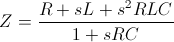## Circuit D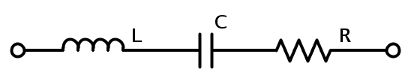Typical usage : capacitor

Impedance :Impedance function to be used for fitting :## Circuit ETypical usage : resonator

Impedance :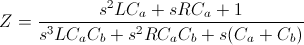Impedance function to be used for fitting :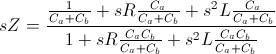References:

  L. Vestling and J. Ankarcrona "A General Small-Signal Series Impedance Extraction Technique," IEEE Microwave and Wireless Components Letters, vol. 12, no. 7, pp. 249-251, July 2002.  L. Vestling "Design and Modeling of High-Frequency LDMOS Transistors," Ph.D. Thesis, ISBN 91-554-5210-8, Uppsala University, Feb.2002.